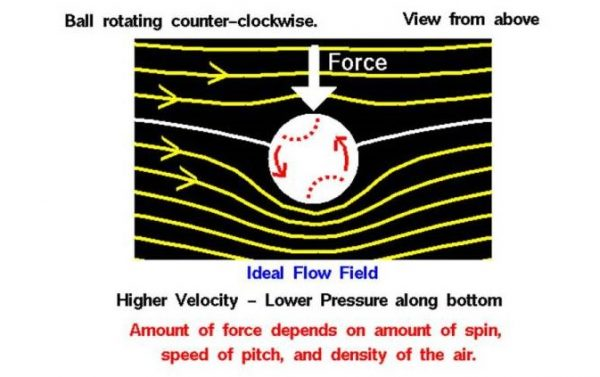# Ideal Flow Around a Spinning BallAll that is necessary to create lift is to turn a flow of air. The airfoil of a wing turns a flow, but so does a spinning ball. The exact details are fairly complex and are given on a separate slide. Summarizing the results, the amount of force generated by a spinning ball depends on the amount of spin, the velocity of the ball, the size of the ball, and the density of the fluid.

### Flow

The figure shows a view of the flow as if we were moving with the ball. The ball appears stationary, and the flow moves from left to right. As the ball spins, the air near the surface of the ball moves with the surface of the ball. If there was no free stream flow and the ball was stopped and spinning, there would be circular flow around the ball which would match the speed of rotation at the surface and die away to nothing far from the ball. When the free stream flow is added to this circular flow, the resulting flow has a net turning and produces a force. On the figure the ball spins counterclockwise, so the free stream flow over the top of the ball is opposed by the circular flow; the free stream flow below the ball is assisted by the circular flow. In the figure we can see that the streamlines around the ball are distorted because of the spinning. The net turning of the flow has produced a downward force.

### Guide to Investigate the Flow around a Spinning Ball:

The left window shows a view of a ball placed in a flow of air. The ball is a foot in diameter and it is moving 100 miles an hour. You can spin the ball by using the slider below the view window or by backspacing over the input box, typing in your new value and hitting the Enter key on the keyboard. On the right is a graph of the lift versus spin. The red dot shows your conditions. Below the graph is the numerical value of the lift. You can display either the lift value (in English or Metric units) or the lift coefficient by using the choice buttons surrounding the output box. select the choice button and select from the drop-menu.

Notice that spinning the ball clockwise generates a positive value for lift, while spinning the ball counter-clockwise generates a negative value of lift. For a clockwise spin, with flow from left to right, the ball would rise. For a counter-clockwise spin, the ball would move downward.

As the force acts on the ball, it is deflected along it’s flight path. If the ball is always spinning, the force is continuous and the ball is deflected along a circular arc. The mathematical details of the ball’s trajectory are given on a separate slide.

### Analysis

Be particularly aware of the simplifying assumptions that have gone into this analysis. The type of flow field shown in the figure is called an ideal flow field. We have produced the ideal flow field by superimposing the flow field from an ideal vortex centered on the ball with a uniform free stream flow. There is no viscosity in this model, no boundary layer on the ball, even though viscosity is the real origin of the circulating flow! In reality, the flow around a spinning ball is very complex because of the boundary layer growth and transition. In addition, the flow off the rear of the ball is normally separated and can even be unsteady. BUT, the simplified model helps us to determine the important parameters and the dependence of the lift force on the value of the parameters. To obtain an accurate value for the force, engineers typically use a lift coefficient that is determined experimentally and accounts for the details that are too complex to model in the analysis.